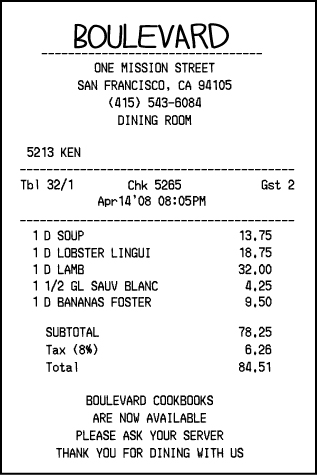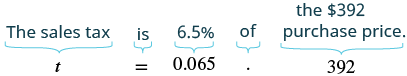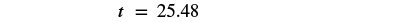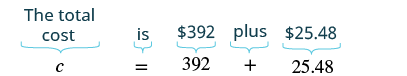Solving Sales Tax Applications

Learning Outcomes

• Calculate total purchase price given sales tax and price
• Calculate sales tax given tax rate and purchase price
• Calculate tax rate given sale price and amount of sales tax

Sales tax and commissions are applications of percent in our everyday lives. To solve these applications, we will follow the same strategy we used in the section on decimal operations. We show it again here for easy reference.

Solve an application

1. Identify what you are asked to find and choose a variable to represent it.
2. Write a sentence that gives the information to find it.
3. Translate the sentence into an equation.
4. Solve the equation using good algebra techniques.
5. Check the answer in the problem and make sure it makes sense.
6. Write a complete sentence that answers the question.

Remember that whatever the application, once we write the sentence with the given information (Step 2), we can translate it to a percent equation and then solve it.

Do you pay a tax when you shop in your city or state? In many parts of the United States, sales tax is added to the purchase price of an item. See the image below.

The sales tax is determined by computing a percent of the purchase price.

To find the sales tax multiply the purchase price by the sales tax rate. Remember to convert the sales tax rate from a percent to a decimal number. Once the sales tax is calculated, it is added to the purchase price. The result is the total cost—this is what the customer pays.

The sales tax is calculated as a percent of the purchase price.Sales Tax

The sales tax is a percent of the purchase price.

$\begin{array}{ccc}\hfill \text{Sales Tax}& =& \text{Tax Rate}\cdot \text{Purchase Price}\hfill \\ \hfill \text{Total Cost}& =& \text{Purchase Price}+\text{Sales Tax}\hfill \end{array}$

example

Cathy bought a bicycle in Washington, where the sales tax rate was $\text{6.5%}$ of the purchase price. What was

ⓐ the sales tax and
ⓑ the total cost of a bicycle if the purchase price of the bicycle was $\text{\392}?$

Solution

 ⓐ Identify what you are asked to find. What is the sales tax? Choose a variable to represent it. Let $t=$ sales tax. Write a sentence that gives the information to find it. The sales tax is $6.5\text{%}$ of the purchase price. Translate into an equation. (Remember to change the percent to a decimal).Simplify.Check: Is this answer reasonable? Yes, because the sales tax amount is less than $10\text{%}$ of the purchase price. Write a complete sentence that answers the question. The sales tax is $\text{\25.48}$.
 ⓑ Identify what you are asked to find. What is the total cost of the bicycle? Choose a variable to represent it. Let $c=$ total cost of bicycle. Write a sentence that gives the information to find it. The total cost is the purchase price plus the sales tax. Translate into an equation.Simplify.Check: Is this answer reasonable? Yes, because the total cost is a little more than the purchase price. Write a complete sentence that answers the question. The total cost of the bicycle is $\text{\417.48}$.

try it

In the following video we show an example of how to calculate the sales tax on an item given the tax rate. Note that it does not include calculating the total cost of the item after tax.

example

Evelyn bought a new smartphone for $\text{\499}$ plus tax. She was surprised when she got the receipt and saw that the tax was $\text{\42.42}$. What was the sales tax rate for this purchase?

TRY IT

Watch this video to see another example of how to find the tax rate for an item given the price after tax, and the original price.